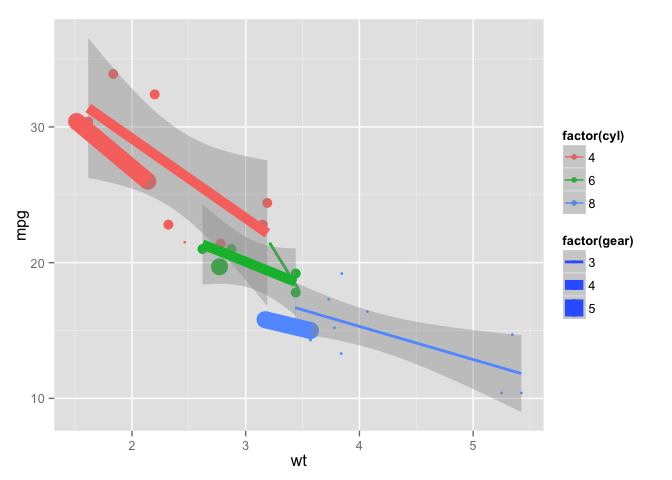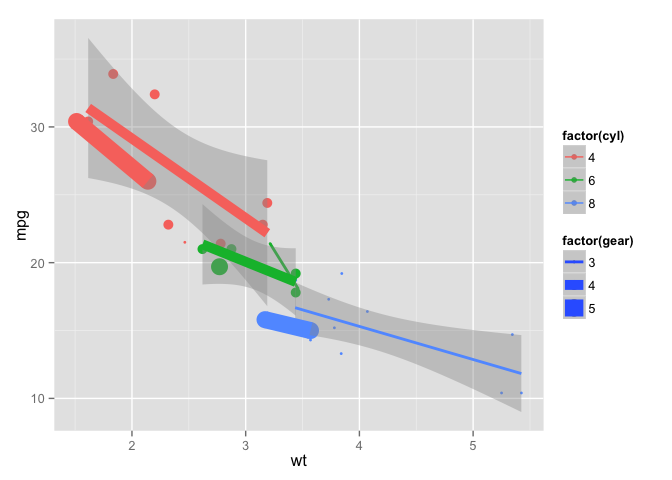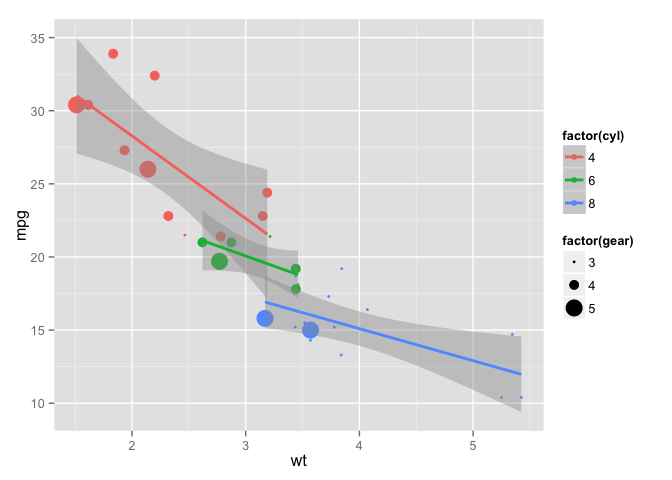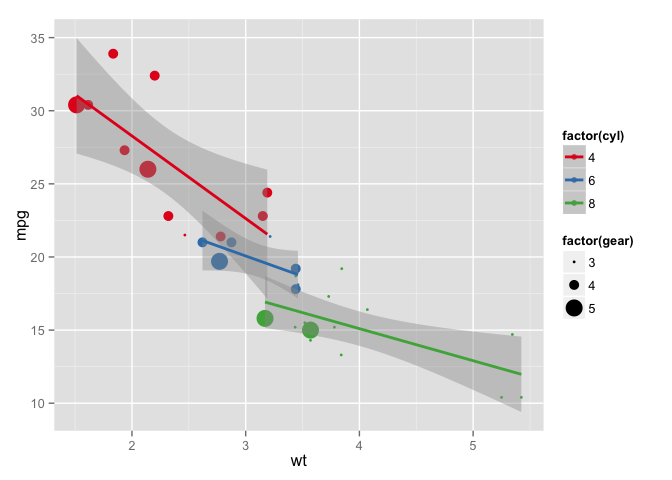qplot: 快速构建图形

qplot(x, y = NULL, ..., data, facets = NULL, margins = FALSE,
geom = "auto", stat = list(NULL), position = list(NULL), xlim = c(NA,
NA), ylim = c(NA, NA), log = "", main = NULL,
xlab = deparse(substitute(x)), ylab = deparse(substitute(y)), asp = NA)
Arguments

x
x values

y
y values

...
other aesthetics passed for each layer

data
data frame to use (optional). If not specified, will create one, extracting vectors from the current environment.

facets
faceting formula to use. Picks facet_wrap or facet_grid depending on whether the formula is one sided or two-sided

margins
whether or not margins will be displayed

geom
character vector specifying geom to use. Defaults to "point" if x and y are specified, and "histogram" if only x is specified.

stat
character vector specifying statistics to use

position
character vector giving position adjustment to use

xlim
limits for x axis

ylim
limits for y axis

log
which variables to log transform ("x", "y", or "xy")

main
character vector or expression for plot title

xlab
character vector or expression for x axis label

ylab
character vector or expression for y axis label

asp
the y/x aspect ratio

library(ggplot2)
p <- ggplot(mtcars,aes(wt, mpg, size=factor(gear)
, color=factor(cyl)))
p + geom_point() + geom_smooth(method='lm')
## Warning in qt((1 - level)/2, df): NaNs produced
## Warning in qt((1 - level)/2, df): NaNs produced
## Warning in qt((1 - level)/2, df): NaNs producedqplot(wt,mpg,size=factor(gear),color=factor(cyl),data=mtcars,
geom=c('point','smooth'), method='lm')
## Warning in qt((1 - level)/2, df): NaNs produced
## Warning in qt((1 - level)/2, df): NaNs produced
## Warning in qt((1 - level)/2, df): NaNs producedp + geom_point() + geom_smooth(method='lm',size=1)qplot(wt,mpg,size=factor(gear),color=factor(cyl),data=mtcars,
geom='point') + geom_smooth(method='lm',size=1) +
scale_color_brewer(palette='Set1')qplot的内容就介绍到这里。下面我们去探索一下ggplot里将数据分组展示的另外一种技术——facet吧！